﻿Fundamental Data Structures: Linked Lists | Vega IT

# Fundamental Data Structures: Linked Lists

Author: Boris Stanojević Categories: Knowledge Base Date 10-May-2021 9 minutes to readWhen we talked about arrays we ended up with a question of a data structure. We concluded that it should not have its size specified in advance, nor it would sometimes have expensive appends.

Before we start talking about this kind of data structure, let us make an overview of the worst-case time complexities of some basic operations on static and dynamic arrays.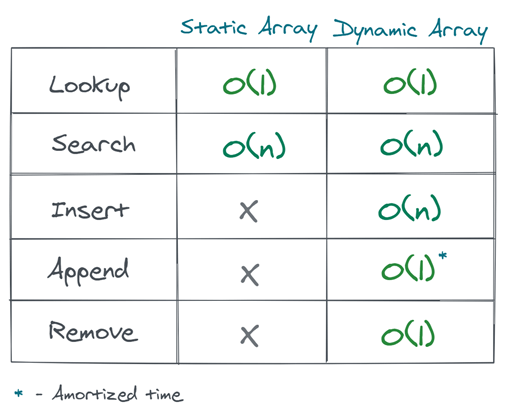### What Are the Basic Operations on Static and Dynamic Arrays?

1. #### Lookup

We can perform a lookup operation in constant time, and this refers to both static and dynamic arrays. This is possible because arrays are indexable.

2. #### Search

Searching for an element with a particular value takes linear time on both static and dynamic arrays. The worst-case scenario could be that the element that we are looking for is at the end, or that it does not exist in the array in the first place.

3. #### Insert

Inserting an element in static arrays does not make much sense since they are fixed in size and cannot expand or shrink dynamically.

Bear in mind that insertion does not mean overwriting an element.

In the case of dynamic arrays, an insertion operation has linear worst-case time complexity because the worst case is that we want to insert an element at the very beginning - prepend.

In that case, we would need to shift all the elements in the array to the right.

Additionally, regardless of whether you insert an element at the very beginning, in the middle, or at the third quarter - the operation still has linear time complexity.

4. #### Append

Appending an element in static arrays does not make much sense just like is the case with insertion.

Appending an element implies adding that element at the very end of an array.

In dynamic arrays, this operation has the amortized time complexity of O(1).

The worst-case scenario, though, would be that the array is full and that we have to expand it which includes allocating a new, bigger array, copying all the n elements into it, and then adding the element at the end of the new array.

Since this would happen relatively rarely - only when the array gets full, we usually speak about amortized time complexity which is O(1).

But, the worst-case time complexity of an append operation is still linear.

5. #### Remove

Removing an element from a static array does not make much sense because we would leave a gap in the memory which other programs cannot use.

In the case of dynamic arrays, a removal operation has linear worst-case time complexity because we would need to shift all subsequent elements to the left in order to fill the gap.

In addition to that, there is also this “problem” that both static and dynamic arrays are static data structures.

Hold on, static arrays are static data structures and dynamic arrays are static data structures as well?

Let us explain.

Static data structures are data structures that require their resources to be allocated when the structures themselves are created.

When creating a static array we have to explicitly define its size:

`int staticArray = new int;`

So, a contiguous block of memory, large enough to store all 10 integers, gets allocated.

When creating a dynamic array:

`DynamicArray<Integer> dynamicArray = new DynamicArray<>();`

Where did the “define its size” part go?

Well, it is abstracted away from us.

When creating a dynamic array, its size is defined implicitly, under the hood. Dynamic arrays are essentially an abstraction over static arrays and the size of these has to be specified somewhere in the code.

This is why both static and dynamic arrays are static data structures - the memory resources are allocated upfront.

A linked list, like an array, is a linear data structure but it is slightly different in how it manages memory.

A linked list allocates additional memory resources during runtime, so there is no need to have a compile-time specified size.

This property is particularly useful in situations where we do not know the number of elements that the data structure will contain in advance.

As elements get added, additional memory resources are allocated, but not upfront.

Take a look at how arrays and linked lists conceptually differ in terms of the memory arrangement.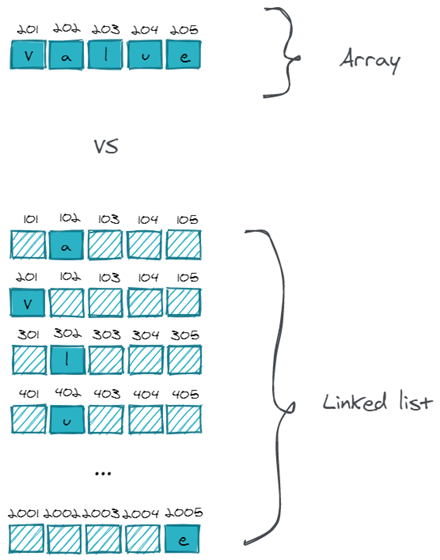Instead of elements being arranged sequentially in the memory, one next to each other, linked lists have their elements possibly scattered all over the memory.

With arrays, the address of the next element can easily be calculated because elements are sequentially ordered in the memory.
We explained that in our article on arrays.

So how does a linked list preserve the idea of an order of the elements, how does it know where the next element is?

Pointers (references) can help us with that.

## The Anatomy Of A Linked List

A linked list consists of a series of things called nodes, or, in some literature, links.

Each node contains two parts:

• The original data - whether it is an integer, string, or some Customer type
• A reference to the next node in the sequence

I will use the term reference instead of pointer since I mostly program in languages like C# and Java.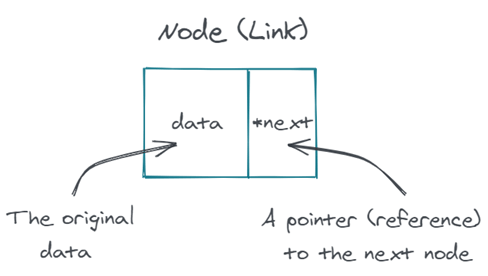So, a linked list is a series of nodes where each node contains data and a reference to the next node.

This is how it conceptually looks like: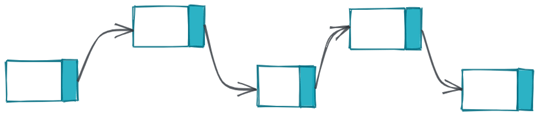Looking at the picture above you might wonder how we know where the end is. The last node in the sequences references nothing.

This means that the value of its next reference is null, None, nil, or any other “non-existing” value - depending on the language we are using.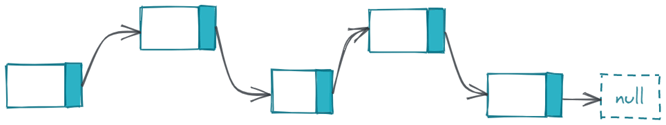Now, how do we know where the beginning is?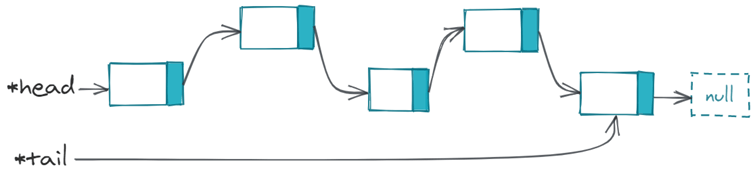A linked list, as a data structure, preserves a reference to the first node, commonly called the head.

It also preserves a reference to the last node, commonly called the tail*.

*To be more precise, the kind of a linked list that, in addition to the head reference, contains the tail reference, is called a double-ended linked list. Not all implementations of a linked list contain the tail reference, but for the purpose of this article, I wanted to focus on this kind of implementation, because it lets us hop to the tail node in a constant time.

This property is useful for performing certain operations efficiently, as well as for the implementations of other data structures such as queues.

There is one more thing I need to mention.

This kind of a linked list is called a singly linked list because each node contains a reference to the next node*.

*Another type of a linked list is a doubly-linked list where nodes contain an additional reference to the previous node.

A doubly-linked list is a bit “heavier” from the perspective of the amount of memory occupied because of the additional reference that each node contains.

However, because each node is aware of the previous node, certain operations can be performed more efficiently than in a singly-linked list.

The concept is rather the same so we will not go deep into the subject of doubly-linked lists.

## Append

Imagine that we wanted to append an element to the linked list.

These are the steps we need to perform:

1. We create a node and set its next reference to null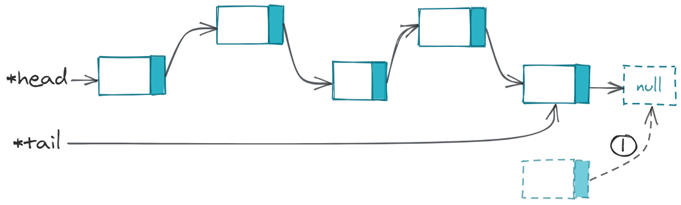2. We set the tail node’s next reference to the newly created node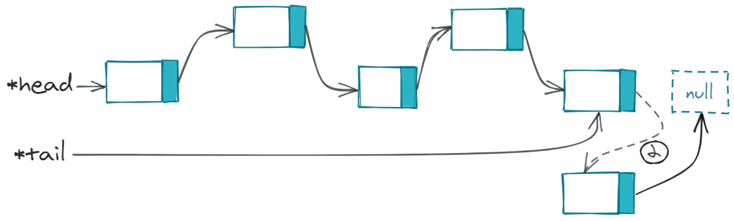3. We make the tail now reference the newly created node as it now becomes the tail node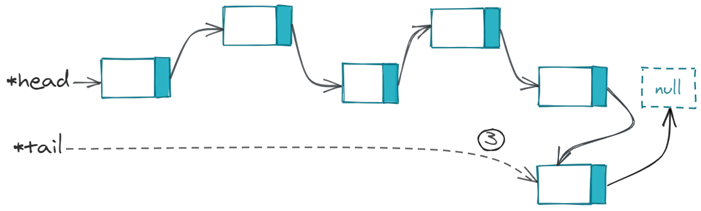And that is it, the list now looks like this: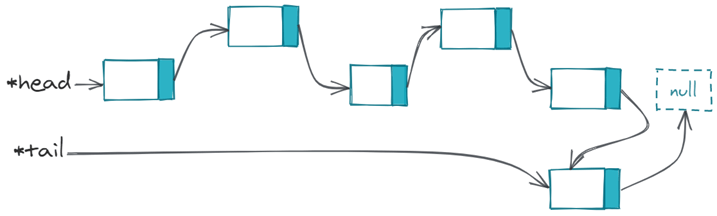As we can see, appending an element to a linked list involves creating a new node and rearranging some references.

We can assume that creating an object and rearranging references are constant-time operations.

We would literally never have to dynamically expand the linked list in order to append an element, as is the case with the worst-case scenario of appending an element to a dynamic array.

We simply append the element, and it gets stored wherever there is free space in the memory.

Appending an element to a linked list has a constant worst-case time complexity - O(1).

## Prepend

Imagine that we wanted to prepend an element to the linked list.

These are the steps that we need to perform:

1. We create a new node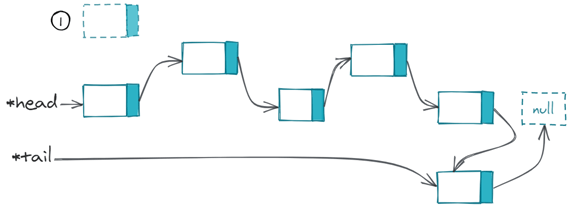2. We set the newly created node’s next reference to what the head is referencing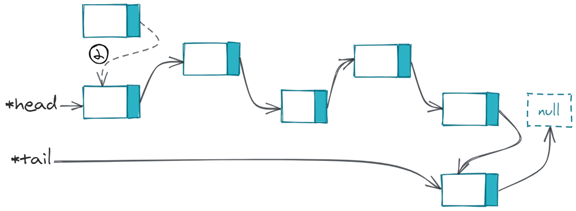3. We make the head now reference the newly created node as it now becomes the head node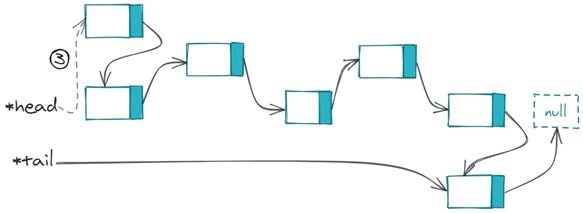The list now looks like this: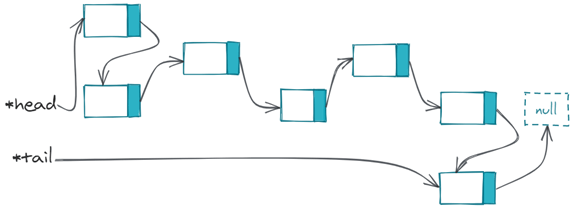As was the case with appending, prepending an element to a linked list also involves creating a new node and rearranging some references.

We would also literally never have to shift all subsequent elements to the right in order to prepend an element, as is the case with dynamic arrays.

We simply prepend the element, and it gets stored wherever there is free space in the memory.

Prepending an element to a linked list has a constant worst-case time complexity - O(1).

## Remove

When removing an element from a linked list there are 2 interesting scenarios to consider:

1. Removing from the beginning
2. Removing from the end

Removing an element from the beginning of a linked list involves rearranging some references and has constant worst-case time complexity - O(1).

Removing an element from the end of a linked list has linear worst-case time complexity - O(n).

Why are the time complexities different ?

In order to remove from the end we would essentially need to set the penultimate node’s next reference to null - which would be a constant time operation.

However, there is no way to get to the penultimate node in constant time. We can get to the tail node because we have reference to it, but we cannot go backwards because each node contains only the next reference.

Therefore, we need to start from the beginning and follow the references until we reach the penultimate node.
This is the reason why removing from the end of a singly linked list is O(n).

Removing From The End In Doubly Linked Lists

I mentioned linked lists where each node, in addition to the next reference, contains an additional previous reference, the so-called doubly linked lists.

This is how a doubly linked list would look like: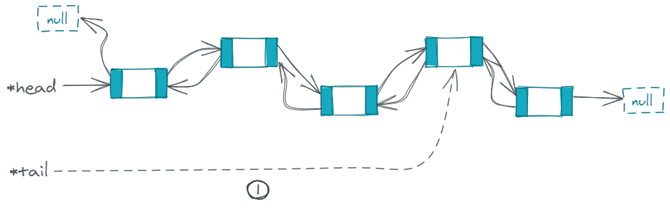I also said that the existence of the reference to the previous node allows us to perform some operations in a more efficient way.

One of those operations is removing the element from the end.

In order to remove the last element from a doubly linked list, these are the steps we would need to perform:

1. Make tail reference the penultimate node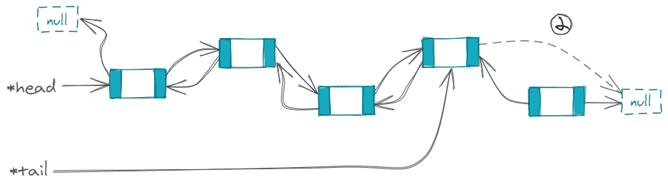2. Make the penultimate node’s next reference be nullSince nothing references the last node any longer, it will eventually be garbage collected - with the assumption that we are talking in the context of programming languages that support automatic memory management.

The list would now look like this: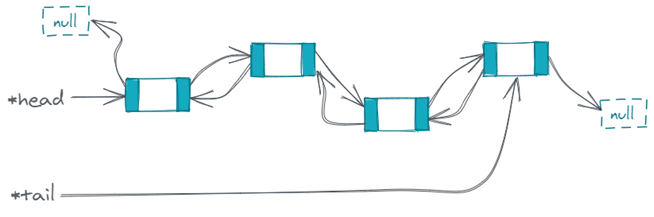And that is it! Removing from the end of a doubly linked list only involved rearranging some references.

Removing an element from the end of a doubly linked list constant worst-case time complexity - O(1).

An interesting fact is that the default implementation of linked list data structure in programming languages such as Java and C# is actually a doubly linked list.

## Lookup and Search

It is worth mentioning that, because of how linked lists manage memory, we cannot use indices to perform lookup operations in constant time.

Due to the indexable nature of arrays, we can lookup an element in a constant time, based on its index - the computer calculates where that element is located in the memory extremely fast.

In linked lists, on the other hand, there is no way to calculate the address of the i-th element.

The only way to get to the i-th element, that is, to the i-th node, is by following the next references.

We start from the head node and follow the next references until we reach the desired node.

Lookups in a linked list have linear worst-case time complexity - O(n).

The same stands for searching a node with a particular value which is not an index.

Searching for a particular value in a linked list has linear worst-case time complexity - O(n).

This is where I would like to end the article.

What if we really need to have constant-time lookups, but there is no no context of indices, as is the case with linked lists?

What if we had a data structure that would allow us to perform constant-time lookup based on some arbitrary value that we commonly refer to as the key?

In the next article I am going to talk about a very interesting data structure - a hash table.

Stay tuned!Boris Stanojević Software Developer

A passionate and lifelong learner. His aspiration is to become a software architect.